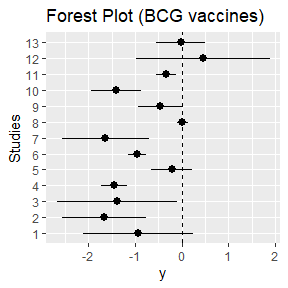# Conducting meta-analysis using MetaStan

## Introduction

To fit meta-analysis models using frequentist methods, there are many R packages available including metafor. On the other hand, Bayesian estimation methods such as Markov chain Monte Carlo (MCMC) are very attractive for meta-analysis, especially because they can be used to fit more complicated models. These include binomial-normal hierarchical models and beta-binomial models which are based on the exact distributional assumptions unlike (commonly used) normal-normal hierarchical model. Another advantage of Bayesian methods to be able to use informative prior distributions for example to regularize heterogeneity estimates in case of low number of studies. Thus, we developed MetaStan which uses Stan (a modern MCMC engine) to fit several pairwise meta-analysis models including binomial-normal hierarchical model and beta-binomial model. This package is also the accompanying package for Günhan, Röver, and Friede (2020).

## Installation

The development version of MetaStan is available on Github (https://github.com/gunhanb/MetaStan) and can be installed using devtools package as follows:

devtools:::install_github("gunhanb/MetaStan")

The BCG trials example is available in the package, and it can be loaded as follows:

library("MetaStan")
data("dat.Berkey1995", package = "MetaStan")
#   Trial Latitude                 publication  r1  r2    n1    n2
# 1     1       44              Aronson (1948)  11   4   139   123
# 2     2       55     Ferguson & Simes (1949)  29   6   303   306
# 3     3       42      Rosenthal et al (1960)  11   3   220   231
# 4     4       52    Hart & Sutherland (1977) 248  62 12867 13598
# 5     5       13 Frimodt-Moller et al (1973)  47  33  5808  5069
# 6     6       44      Stein & Aronson (1953) 372 180  1451  1541

Additional information can be obtained by typing ?dat.Berkey1995 (for any dataset and function in the package).

We can visualize individual log odds ratio estimates plot using ggplot2 as follows:

library(ggplot2)
# Calculating log odds ratios and variances from data
logodds <- function(x) log((x * (x - x))/((x - x) * x))
stdes   <- function(x) sqrt(1/x + 1/(x - x) + 1/x + 1/(x - x))
r_ind   <- apply(cbind(dat.Berkey1995$r2, dat.Berkey1995$n2,
dat.Berkey1995$r1, dat.Berkey1995$n1), 1, logodds)
se_ind  <- apply(cbind(dat.Berkey1995$r2, dat.Berkey1995$n2,
dat.Berkey1995$r1, dat.Berkey1995$n1), 1, stdes)
lower95_ind <- r_ind + qnorm(.025) * se_ind
upper95_ind <- r_ind + qnorm(.975) * se_ind
# Comparison of the results
trials  <- c("1", "2" ,"3", "4", "5", "6", "7", "8", "9", "10", "11", "12", "13")
trials <- ordered(trials, levels = trials)

d <- data.frame(x = trials,
y = r_ind,
ylo = lower95_ind,
yhi = upper95_ind)
forest.plot <- ggplot(d, aes(x = x, y = y, ymin = ylo, ymax = yhi)) +
geom_pointrange() +
coord_flip() +
geom_hline(aes(yintercept=0), lty = 2) +
xlab("Studies") +
ggtitle("Forest Plot (BCG vaccines)")

plot(forest.plot)## Fitting a binomial-normal hierarchical model

metastan is the main fitting function of this package. The main computations are executed via the rstan package’s sampling function. We can fit the binomial-normal hierarchical model (Günhan, Röver, and Friede, 2020) using a weakly informative prior for treatment effect as follows:

 data('dat.Berkey1995', package = "MetaStan")
## Fitting a Binomial-Normal Hierarchical model using WIP priors
data('dat.Berkey1995', package = "MetaStan")
## Fitting a Binomial-Normal Hierarchical model using WIP priors
dat_MetaStan <- create_MetaStan_dat(dat = dat.Berkey1995,
armVars = c(responders = "r", sampleSize = "n"))

meta.BCG.stan  <- meta_stan(data = dat_MetaStan,
likelihood = "binomial",
mu_prior = c(0, 10),
theta_prior = c(0, 100),
tau_prior = 0.5,
tau_prior_dist = "half-normal")

Convergence diagnostics, very conveniently, obtained using shinystan package as follows:

library("shinystan")
## Firstly convert "stan" object to a "shinystan" object
bnhm1.BCG.shinystan = as.shinystan(meta.BCG.stan\$fit)
launch_shinystan(bnhm1.BCG.shinystan)

A simple summary of the fitted model is given by print option:

print(meta.BCG.stan)
# Meta-analysis using MetaStan
#
# Maximum Rhat: 1.01
# Minimum Effective Sample Size: 950
#
# mu prior: Normal(0,10)
# theta prior: Normal(0,100)
# tau prior:half-normal(0.5)
#
# Treatment effect (theta) estimates
#  mean  2.5%   50% 97.5%
# -0.76 -1.16 -0.76 -0.38
#
# Heterogeneity stdev (tau)
#      Mean Lower  50% Upper
# [1,]  0.6  0.35 0.58  0.91

Note that this model corresponds to Model 4 in Jackson et al (2018).

Please see Günhan, Röver, and Friede (2020) and Jackson et al (2018) for complete model descriptions.